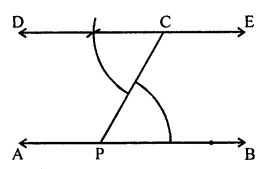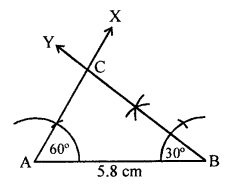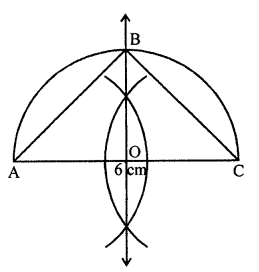## ML Aggarwal Class 7 Solutions for ICSE Maths Chapter 13 Practical Geometry Check Your Progress

Question 1.
State whether the following statements are true or false. Justify your answer.
(i) A triangle with lengths of sides 2.5 cm, 3 cm, and 6 cm can be constructed.
(ii) A triangle DEF with EF = 7.2 cm, m∠E = 110° and m∠F = 80° can be constructed.
(iii) If the measure of an acute angle and the length of the hypotenuse of a right-angled triangle are given, then the triangle can be constructed.
Solution:
(i) We know that in a triangle,
the sum of its any two sides is greater than its third side.
Therefore, a triangle with sides 2.5 cm, 3 cm and 6 cm.
2.5 + 3 = 5.5 < 6 cm
This triangle is not possible.
(ii) In triangle DEF with side EF = 7.2 cm
and m∠E =110° and m∠E = 80°.
The sum of these two angles is 110° + 80° = 190°
which is not possible as a triangle has a sum of 180°.
(iii) If the measure of an acute angle and length of the
hypotenuse in a right-angled triangle is given.
Yes this triangle can be constructed,
Measure of third acute angle = 180° – 90° one acute angle
We are given one side i.e., hypotenuse and its ends angles
which is known as ASA criterion.

Question 2.
Draw a line AB and take a point C outside it. Through C, draw a line parallel to AB by using the concept of equal corresponding angles.
Solution:
Steps of construction:

1. Draw a line AB and take a point C outside it.
2. Take a point P on AB and join PC.
3. Construct ∠PCD = ∠CPB and produce C to D and C to E.
Then, DE is parallel to AB.Question 3.
Draw a triangle PQR with PQ = 4 cm, QR = 3.5 cm and PR = 4 cm. What type of triangle is this?
Solution:
Steps of construction:

1. Draw a line segment QR = 3.5 cm.
2. At Q and R as centers and radius 4 cm,
draw arcs intersecting each other at P.
3. Join PQ and PR.
∆PQR is the required triangle.Question 4.
Construct a triangle ABC with BC = 7.5 cm, AC = 5 cm and m∠C = 60° by using ruler and compasses only.
Solution:
Steps of construction :

1. Draw a line segment BC = 7.5 cm.
2. At C, draw a ray CX making an angle of 60°
and cut off CA = 5 cm.
3. Join AB.
∆ABC is the required triangle.Question 5.
Construct a triangle ABC, given m∠A = 60°, m∠B = 30° and AB = 5.8 cm by using ruler and compasses only.
Solution:
Steps of construction:

1. Draw a line segment AB = 5.8 cm.
2. At A draw a ray AX making an angle of 60° and at B,
a ray BY making an angle of 30° which intersect each other at C.
∆ABC is the required triangle.Question 6.
Construct an isosceles right angled triangle ABC, with m∠ABC = 90° and AC = 6 cm.
Solution:
Steps of construction:

1. Draw a line segment AC = 6 cm.
2. Draw its perpendicular bisector which intersects AC at O.
3. With centre O and AC as diameter, draw a semicircle.
4. The perpendicular bisects intersect the semicircle at B.
5. Join BA and BC.
∆ABC is the required triangle.